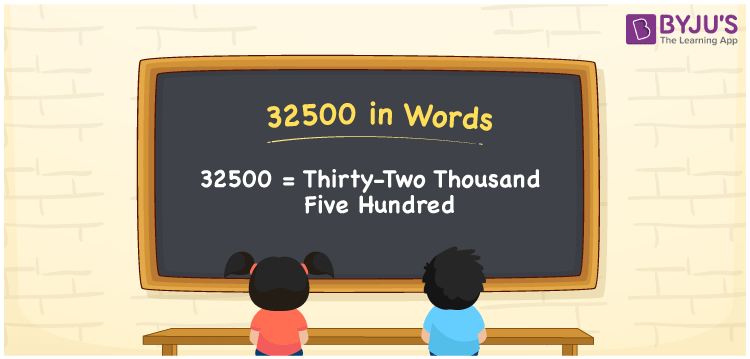# 32500 in Words

32500 in words is written as “Thirty-two thousand five hundred”. For example, Rs. 32500 is given by Rupees “Thirty-two thousand five hundred only” in a bank cheque. In Mathematics, 32500 is also a cardinal number, that is to represent the quantity or value of something. Let us see in this article how to write 32500 in words using place value method.

 32500 in Words Thirty-two thousand five hundred Thirty-two thousand five hundred in Numbers 32500

## 32500 in English Words## How to Write 32500 in Words?

32500 is a five-digit number that can be written in words, by knowing the position of each digit in the number. This can be done using a place value chart.

 Ten thousands Thousands Hundreds Tens Ones 3 2 5 0 0

We can see, from the above table, the positions of digits are:

• 3 → Ten thousands
• 2 → Thousands
• 5 → Hundreds
• 0 → Tens
• 0 → Ones

Hence, when we read the number from right to left, it is given by Thirty-two thousand five hundred. Learn more about numbers in words at BYJU’S.

### Expanded Form of 32500

We can write the expanded form as:

3 × Ten thousand + 2 × Thousand + 5 × Hundred + 0 × Ten + 0 × One

= 3 × 10000 + 2 × 1000 + 5 × 100 + 0 × 10 + 0 × 1

= 30000 + 2000 + 500 + 0 + 0

= 32500

= Thirty-two thousand five hundred

32500 is the natural number that is succeeded by 32499 and preceded by 32501. Learn more about the number 32500 below:

• 32500 in Words – Thirty-two thousand five hundred
• Is 32500 an odd number? – No
• Is 32500 an even number? – Yes
• Is 32500 a perfect square number? – No
• Is 32500 a perfect cube number? – No
• Is 32500 a prime number? – No
• Is 32500 a composite number? – Yes

## Frequently Asked Questions on 32500 in words

Q1

### What is 32500 in words?

32500 in words is given by Thirty-two thousand five hundred.
Q2

### What is the place value of 5 in 32500?

The place value of 5 in 32500 is Hundreds.
Q3

### How to spell 32500 in English words?

32500 in English is spelt as Thirty-two thousand five hundred